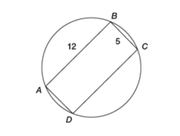• 7 CATs FREE!
If you earn 100 Forum Points

Engage in the Beat The GMAT forums to earn
100 points for \$49 worth of Veritas practice GMATs FREEVERITAS PRACTICE GMAT EXAMS
Earn 10 Points Per Post
Earn 10 Points Per Thanks
Earn 10 Points Per Upvote

## If rectangle ABCD is inscribed in the circle above, what is

##### This topic has expert replies
Master | Next Rank: 500 Posts
Posts: 394
Joined: 02 Jul 2017
Thanked: 1 times
Followed by:4 members

### If rectangle ABCD is inscribed in the circle above, what is

by AbeNeedsAnswers » Fri May 03, 2019 5:54 pm

00:00

A

B

C

D

E

## Global StatsIf rectangle ABCD is inscribed in the circle above, what is the area of the circular region?

A) 36.00Ï€
B) 42.25Ï€
C) 64.00Ï€
D) 84.50Ï€
E) 169.00Ï€

B

Source: Official Guide 2020

### GMAT/MBA Expert

GMAT Instructor
Posts: 14926
Joined: 08 Dec 2008
Location: Vancouver, BC
Thanked: 5254 times
Followed by:1265 members
GMAT Score:770
by Brent@GMATPrepNow » Sat May 04, 2019 6:01 am
AbeNeedsAnswers wrote:If rectangle ABCD is inscribed in the circle above, what is the area of the circular region?

A) 36.00Ï€
B) 42.25Ï€
C) 64.00Ï€
D) 84.50Ï€
E) 169.00Ï€

B

Source: Official Guide 2020
Draw a line connecting points A and C.An important circle property (see video below for more info) tells us that, if we have a 90-degree inscribed angle, then that angle must be containing ("holding") the DIAMETER of the circle.
So, we know that AC = the diameter of the circle.

To find the hypotenuse of the red triangle, we can EITHER apply the Pythagorean Theorem OR recognize that 5 and 12 are part of the Pythagorean triplet 5-12-13With either approach, we learn that AC = 13

IMPORTANT: if the diameter (AC) is 13, then the radius = 13/2 = 6.5

What is the area of the circular region?
Area of circle = Ï€rÂ²
So, area = Ï€(6.5Â²)
= 42.25Ï€

Cheers,
Brent
Brent Hanneson - Creator of GMATPrepNow.com
If you enjoy my solutions, I think you'll like my GMAT prep courseWatch these video reviews of my course
And check out these free resources

Legendary Member
Posts: 1632
Joined: 29 Oct 2017
Followed by:5 members
by swerve » Sat May 04, 2019 10:36 am
The rectangle is equally placed on the center of the circle.

Let $$O$$ be the center of the circle.

And $$X$$ be the midpoint of $$AB$$.

Then $$OX = \frac{5}{2}$$ and $$XB = \frac{12}{2} = 6$$

Triangle $$OXB$$ is a right angles triangle.

$$OB = radius = \sqrt{6^2+2.5^2}=\sqrt{42.5}$$

Area $$= \pi \cdot r^2 = 42.5\pi$$

Hence, __B__

### GMAT/MBA Expert

Elite Legendary Member
Posts: 10347
Joined: 23 Jun 2013
Location: Palo Alto, CA
Thanked: 2867 times
Followed by:506 members
GMAT Score:800
by Rich.C@EMPOWERgmat.com » Wed May 22, 2019 8:46 am
Hi All,

We're told that a rectangle with sides of 5 and 12 is inscribed in the circle. We're asked for the area of the circular region. This question is based on a number of math patterns that can help you to save time answering the question.

First, any square or rectangle that is inscribed in a circle will have a diagonal that IS the diameter of the circle. Second, when splitting a rectangle or square across its diagonal, you'll form two right triangles. With the given side lengths of the rectangle (5 and 12), we have a 5/12/13 right triangle, so we know the diameter of the circle is 13. The radius is half the diameter... radius = 6.5

Area of a circle = (Ï€)(R^2) = (Ï€)(6.5^2)

At this point, you don't actually have to calculate the value. Since 6^2 = 36 and 7^2 = 49, we know the correct answer has to be between 36Ï€ and 49Ï€. There's only one answer that fits...